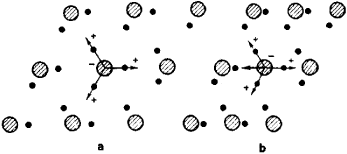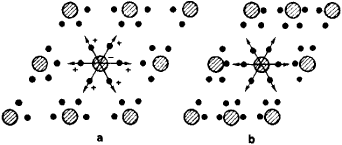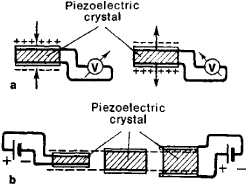# Piezoelectricity

Also found in: Dictionary, Thesaurus, Medical, Wikipedia.
Related to Piezoelectricity: piezoelectric effect

## piezoelectric effect

, piezoelectricity
Physics
a. the production of electricity or electric polarity by applying a mechanical stress to certain crystals
b. the converse effect in which stress is produced in a crystal as a result of an applied potential difference

## Piezoelectricity

Electricity, or electric polarity, resulting from the application of mechanical pressure on a dielectric crystal. The application of a mechanical stress produces in certain dielectric (electrically nonconducting) crystals an electric polarization (electric dipole moment per cubic meter) which is proportional to this stress. If the crystal is isolated, this polarization manifests itself as a voltage across the crystal, and if the crystal is short-circuited, a flow of charge can be observed during loading. Conversely, application of a voltage between certain faces of the crystal produces a mechanical distortion of the material. This reciprocal relationship is referred to as the piezoelectric effect. The phenomenon of generation of a voltage under mechanical stress is referred to as the direct piezoelectric effect, and the mechanical strain produced in the crystal under electric stress is called the converse piezoelectric effect. See Polarization of dielectrics

The necessary condition for the piezoelectric effect is the absence of a center of symmetry in the crystal structure. Of the 32 crystal classes, 21 lack a center of symmetry, and with the exception of one class, all of these are piezoelectric. Hydrostatic pressure produces a piezoelectric polarization in the crystals of those 10 classes that show pyroelectricity in addition to piezoelectricity. See Crystallography;, Pyroelectricity

#### Molecular theory

Quantitative theories based on the detailed crystal structure are very involved. Qualitatively, however, the piezoelectric effect is readily understood for simple crystal structures. The illustration shows this for a particular cubic crystal, zincblende (ZnS). Every Zn ion is positively charged and is located in the center of a regular tetrahedron ABCD, the corners of which are the centers of sulfur ions, which are negatively charged. When this system is subjected to a shear stress in the xy plane, the edge AB, for example, is elongated, and the edge CD of the tetrahedron becomes shorter. Consequently, these edges are no longer equivalent, and the Zn ion will be displaced along the z axis, thus giving rise to an electric dipole moment. The dipole moments arising from different octahedrons sum up because they all have the same orientation with respect to the axes x, y, and z.

#### Applications

The sharp resonance curve of a piezoelectric resonator makes it useful in the stabilization of the frequency of radio oscillators. Quartz crystals are used almost exclusively in this application. In vacuum-tube oscillators, the crystal generally is part of the feedback circuit. Selective band-pass filters with low losses can be built by using piezoelectric resonators as circuit elements. A synthetic piezoelectric crystal which is often substituted for quartz in this application is ethylene diamine tartrate.

Piezoelectric materials are used extensively in transducers for converting a mechanical strain into an electrical signal. Such devices include microphones, phonograph pickups, vibration-sensing elements, and the like. The converse effect, in which a mechanical output is derived from an electrical signal input, is also widely used in such devices as sonic and ultrasonic transducers, headphones, loudspeakers, and cutting heads for disk recording.

#### Piezoelectric materials

The principal piezoelectric materials used commercially are crystalline quartz and rochelle salt, although the latter is being superseded by other materials, such as barium titanate. Quartz has the important qualities of being a completely oxidized compound (silicon dioxide), and is almost insoluble in water. Therefore, it is chemically stable against changes occurring with time. It also has low internal losses when used as a vibrator. Rochelle salt has a large piezoelectric effect, and is thus useful in acoustical and vibrational devices where sensitivity is necessary, but it decomposes at high temperatures (131°F or 55°C) and requires protection against moisture. Barium titanate provides lower sensitivity, but greater immunity to temperature and humidity effects. Other crystals that have been used for piezoelectric devices include tourmaline, ammonium dihydrogen phosphate (ADP), and ethylenediamine tartrate (EDT).

## Piezoelectricity

the phenomenon that describes both the polarization that arises in a dielectric as a result of an applied mechanical stress and the mechanical strain that occurs as a result of an applied electric field. The former is known as the direct piezoelectric effect, and the latter as the converse piezoelectric effect. Direct and converse effects can both be observed in the same piezoelectric crystals.

The first detailed investigation of piezoelectric effects was conducted in 1880 by the brothers Jacques and Pierre Curie on quartz crystals. Later on, piezoelectric properties were found in more than 1,500 substances, including the widely used Rochelle salt and barium titanate.Figure 1. Planar model of a crystal lacking a center of symmetry: (a) The centers of gravity of the positive and negative charges coincide; the arrows indicate the separate electric dipole moments of a group of charges. (b) A compressive stress changes the distances between the charges but does not change the angles; the horizontal arrow pointing to the left represents the resultant electric dipole moment of a group of charges.

The piezoelectric properties of a crystal are associated with its structure. Pyroelectrics, or spontaneously polarized dielectrics, also exhibit these properties. Mechanical strain in a pyroelectric will change the magnitude of its spontaneous polarization; thus, a direct piezoelectric effect is observed. Piezoelectric effects are also observed in certain nonpyroelectric substances, such as quartz. It is valid to state, as a general principle, that crystals having a center of symmetry cannot exhibit piezoelectric effects. This is because the crystal’s center of symmetry remains intact during strain, and as long as a center of symmetry exists, there can be no polarization (Figures 1 and 2). Polarization may be ruled out along certain directions or with certain strains if the crystal is symmetric along a given axis or in a given plane.Figure 2. Planar model of a crystal having a center of symmetry: (a) not under compressive stress and (b) under compressive stress

In a given crystal, the quantitative piezoelectric properties are given by the piezoelectric constants and piezoelectric strain coefficients. These are coefficients of proportionality that relate electrical quantities, such as the intensity of the electric field E or the polarization P, to mechanical quantities, such as mechanical stress σ or relative strain u. In the equation P = d σ, the coefficient d is one of the piezoelectric constants. Since any arbitrary mechanical stress can be represented as a total of six independent stresses and since the polarization vector P has three independent components, there can be 18 possible piezoelectric constants. The symmetry of the crystal, however, limits the number of constants that are independent and differ from zero. The magnitude of d depends on the experimental conditions.Figure 3. Piezoelectric effects: (a) direct piezoelectric effect, where a compressive or tensile stress exerted on a piezoelectric crystal leads to the appearance of a potential difference, and (b) converse piezoelectric effect, where, depending on the sign of the applied potential difference, the crystal experiences a compressive or tensile strain

It has one value, d, when the charge on the capacitor plates is kept equal to zero (Figure 3) and a different value, d’, when the capacitor plates are short-circuited; that is, E = 0. For this reason, it is expedient to rewrite the relationship P = d σ as, for example, P = d’ σ + KE. The values of d and d’ are related by the expression d’ = d∈, where ∈ is the dielectric constant of the crystal.

In relations such as P = ru + κ’E, U = S’σ + hP, and u = S’σ + hE, the coefficients r, g, and h are also called piezoelectric constants. All piezoelectric constants d, r, g, and h are interrelated; hence, in describing the piezoelectric properties of a crystal, it is sufficient to state only one constant, such as d. A typical value of the piezoelectric constant d for quartz in the cgs electrostatic system is 3 × 10-8. The piezoelectric constants of ferroelectrics can attain much higher values because of their domains, which can be modified during strain, and their high dielectric constants.

Piezoelectric crystals are widely used in among other fields, engineering, acoustics, and radiophysics. Their use is based on conversion of electrical signals to mechanical signals, and vice versa. Piezoelectric crystals are also used in the resonators that act as oscillator components, in filters, and in various types of transducers and sensors.

### REFERENCES

Cady, W. P’ezoelektrichestvo i ego prakticheskoe primenenie. Moscow, 1949. (Translated from English.)
Mason, W. P’ezoelektricheskie kristally i ikh primenenie v ul’traakustike. Moscow, 1952. (Translated from English.)
Berlincourt, T. [et al.]. “P’ezoelektricheskie p’ezomagnitnye materialy i ikh primenenie v preobrazovateliakh.” In Fizicheskaia akustika, vol. 1, part A. Edited by W. Mason. Moscow, 1966. (Translated from English.)

A. P. LEVANIUK and D. G. SANNIKOV

## piezoelectricity

[pē¦ā·zō·ə‚lek′tris·əd·ē]
(solid-state physics)
Electricity or electric polarization resulting from the piezoelectric effect.
References in periodicals archive ?
"The Piezoelectricity of Poly(Vinylidene Fluoride)." Japanese Journal of Applied Physics.
Piezoelectricity is related to the generation of opposite electric charges on the surfaces of a crystal, when it is subjected to directional stress or under hydrostatic stresses .
Professor Tofail Syed from the University's Physics Department said: "Crystals are the gold-standard for measuring piezoelectricity in non-biological materials.
Starting from the governing equations of piezoelectricity and following the derivation presented by Rovenski et al.
For langasite family, there are many investigations about the relationship between piezoelectricity and chemical composition.
As a result, current flexoelectric devices are only minimal variations of configurations conceived within the uniform-strain mindset of piezoelectricity. Our main objective in this proposal is to develop an advanced computational infrastructure to quantify flexoelectricity in solids, focusing on continuum models but also exploring multiscale aspects.
In addition, when measuring CVP, we used a U-tube manometer instead of a piezoelectricity transducer.
A commercial 6125B type quartz crystal piezoelectricity sensor made by Kistler is selected, whose measurement range is 0~25MPa and accuracy is 150pc/MPa.
[20.] Dal Corso A, Posternak M, Resta R, Baldereschi A (1994) Ab initio study of piezoelectricity and spontaneous polarization in ZnO.
Yang, Saint Venant's principle in linear piezoelectricity, Journal of Elasticity 38, (1995), pp.
To create the piezoelectric properties in the electrospun PVDF nanofiber web, the [beta]-phase crystalline should be formed which is the one with the most effective piezoelectricity. Hence, X-ray diffraction (XRD) of the samples with and without LiCl was performed to evaluate the formation of this phase.
The variational equations for linear piezoelectricity are presented in this section.

Site: Follow: Share:
Open / Close